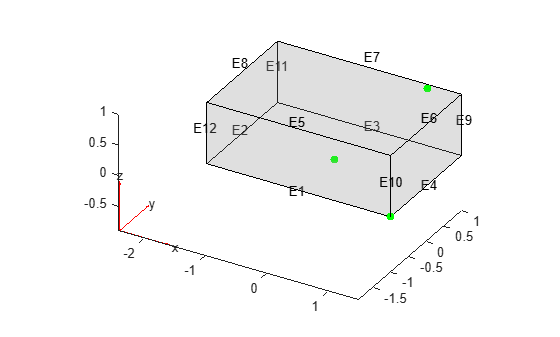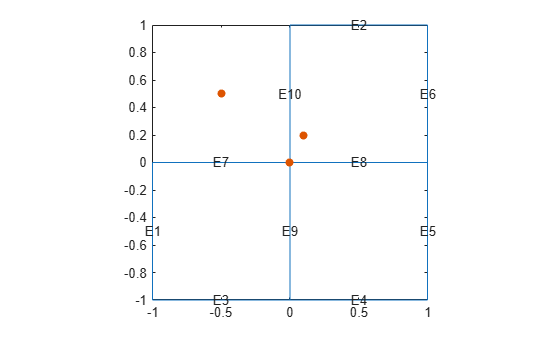# nearestEdge

Find edges nearest to specified point

Since R2021a

## Syntax

``EdgeID = nearestEdge(g,Coords)``

## Description

example

````EdgeID = nearestEdge(g,Coords)` finds edges nearest to the point with the coordinates `Coords`.```

## Examples

collapse all

Find edges of a block nearest to the specified points.

Create a block geometry.

`gm = multicuboid(3,2,1)`
```gm = DiscreteGeometry with properties: NumCells: 1 NumFaces: 6 NumEdges: 12 NumVertices: 8 Vertices: [8x3 double] ```

Plot the geometry with the edge labels. Add the points with the coordinates (0 0 0), (1 0.9 1), and (1.5 -1 0) to the plot.

```pdegplot(gm,"EdgeLabels","on","FaceAlpha",0.2) hold on scatter3([0 1 1.5],[0 0.9 -1],[0 1 0],"filled","MarkerFaceColor","g")```Find edges closest to the points with the coordinates (0 0 0), (1 0.9 1), and (1.5 -1 0). If several edges are equally close (within the tolerance) to the point, `nearestEdge` returns the ID of one of the edges.

`edgeIDs = nearestEdge(gm,[0 0 0; 1 0.9 1; 1.5 -1 0])`
```edgeIDs = 1×3 1 7 1 ```

Find edges of the L-shaped membrane nearest to the specified points.

Create a model and include this geometry. The geometry of the L-shaped membrane is described in the file `lshapeg`.

```model = createpde(); gm = geometryFromEdges(model,@lshapeg)```
```gm = AnalyticGeometry with properties: NumCells: 0 NumFaces: 3 NumEdges: 10 NumVertices: 8 Vertices: [8x2 double] ```

Plot the geometry with the edge labels. Add the points with the coordinates (0 0), (0.1 0.2), and (-0.5 0.5) to the plot.

```pdegplot(gm,"EdgeLabels","on") hold on scatter([0 0.1 -0.5],[0 0.2 0.5],"filled")```Find edges closest to the points with the coordinates (0 0), (0.1 0.2), and (-0.5 0.5). If several edges are equally close (within the tolerance) to the point, `nearestEdge` returns the ID of one of the edges.

`edgeIDs = nearestEdge(gm,[0 0; 0.1 0.2; -0.5 0.5])`
```edgeIDs = 1×3 7 10 10 ```

## Input Arguments

collapse all

Geometry, specified as an `fegeometry` object, a `DiscreteGeometry` object, or an `AnalyticGeometry` object. See `fegeometry`, DiscreteGeometry Properties, and AnalyticGeometry Properties.

Coordinates of the points, specified as an `N`-by-2 or `N`-by-3 numeric matrix for a 2-D or 3-D geometry, respectively. Here, `N` is the number of points.

Data Types: `double`

## Output Arguments

collapse all

IDs of edges nearest to the specified point, returned as a positive number or a vector of positive numbers.

## Version History

Introduced in R2021a

expand all